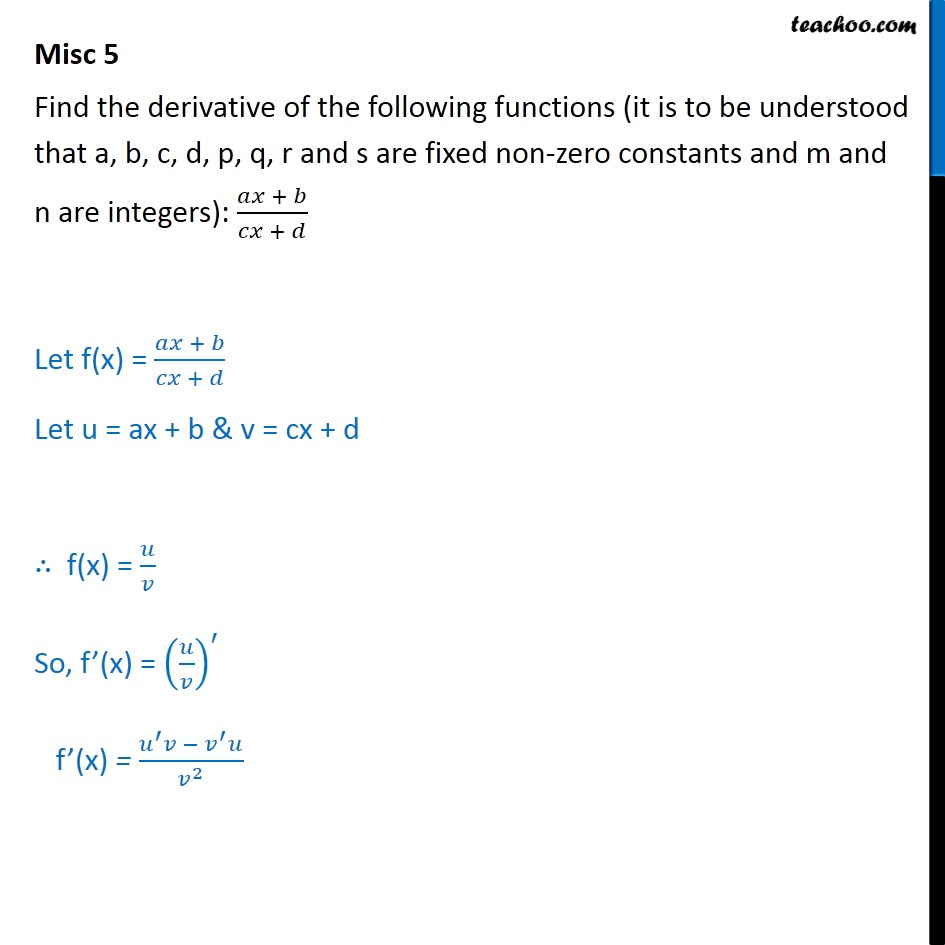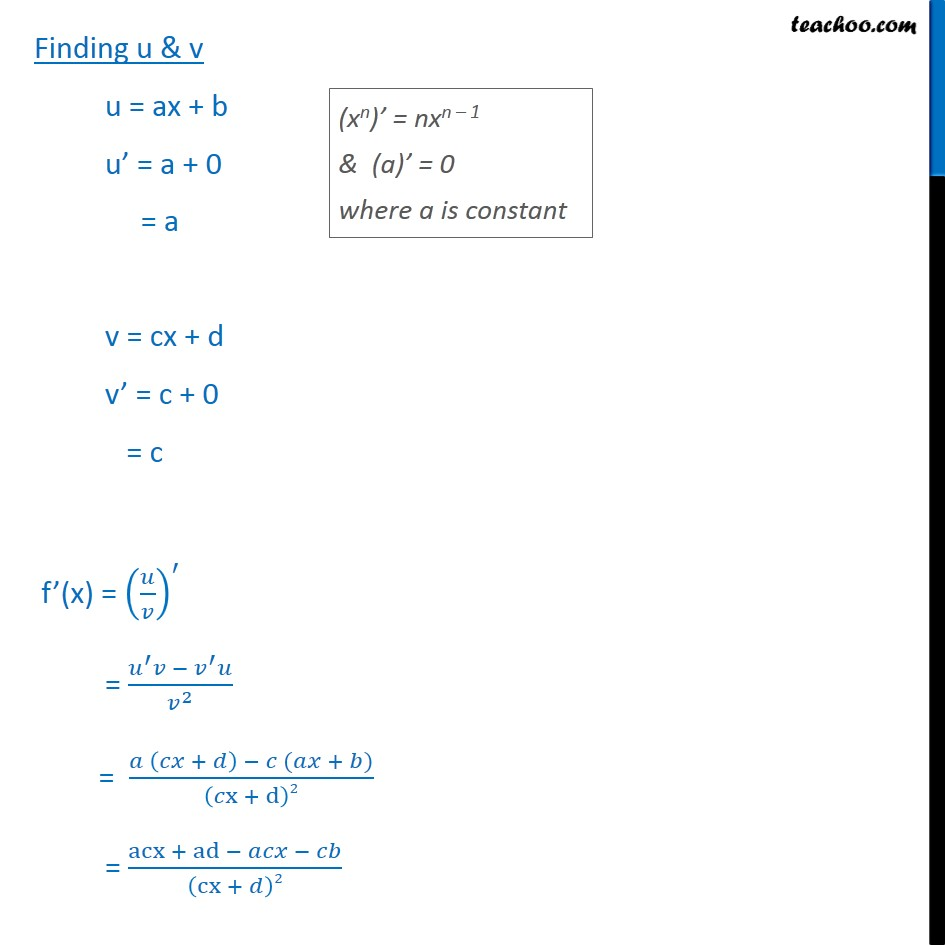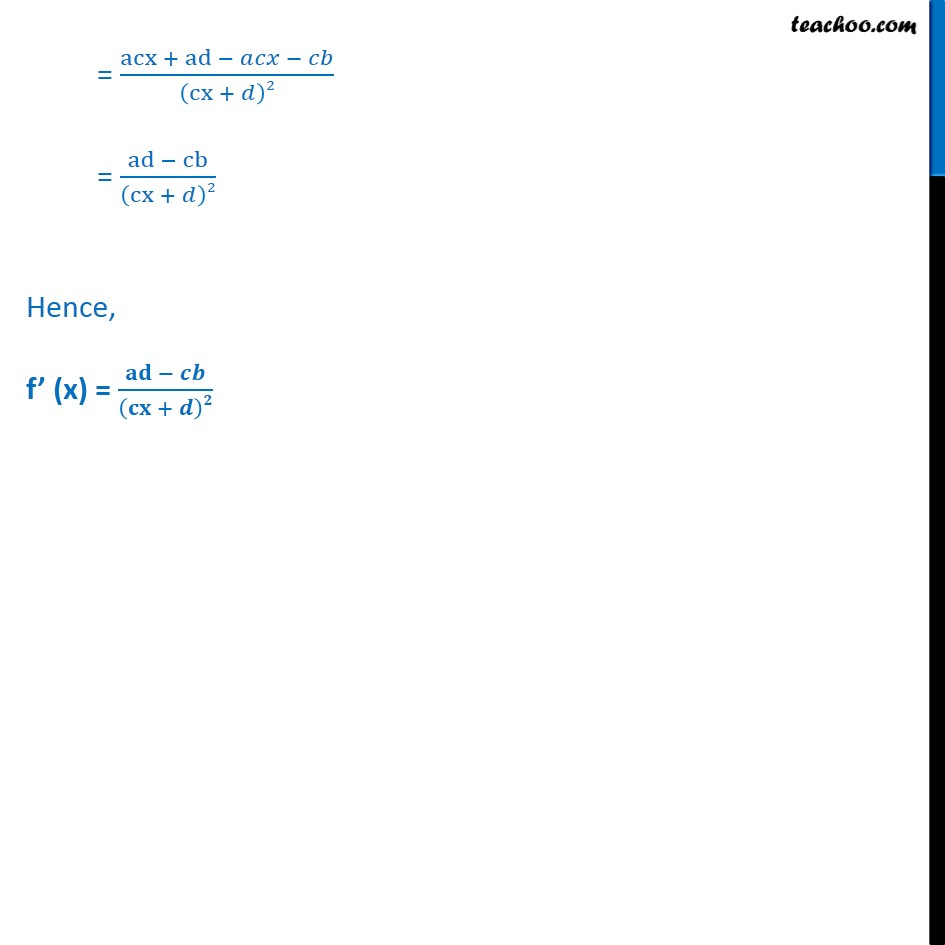Miscellaneous

Chapter 12 Class 11 Limits and Derivatives
Serial order wiseLearn in your speed, with individual attention - Teachoo Maths 1-on-1 Class

### Transcript

Misc 5 Find the derivative of the following functions (it is to be understood that a, b, c, d, p, q, r and s are fixed non-zero constants and m and n are integers): + + Let f(x) = + + Let u = ax + b & v = cx + d f(x) = So, f (x) = f (x) = 2 Finding u & v u = ax + b u = a + 0 = a v = cx + d v = c + 0 = c f (x) = = 2 = + ( + ) x + d 2 = acx + ad cx + 2 = acx + ad cx + 2 = ad cb cx + 2 Hence, f (x) = +